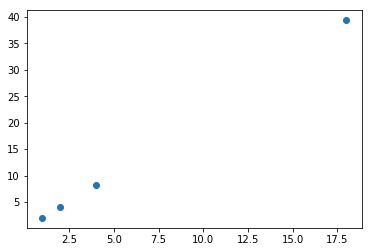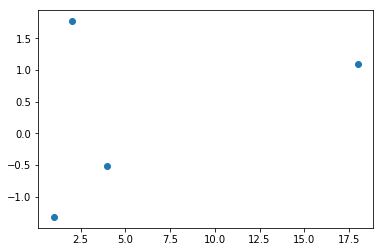### Note: This is a mirror of the official fast.ai course nb for the dsnet meetup. Please check the course repo for the latest notebooks

In [ ]:
%load_ext autoreload
%autoreload 2

%matplotlib inline

In [ ]:
import torch
import matplotlib.pyplot as plt

### Callbacks

#### Callbacks as GUI events

In [ ]:
import ipywidgets as widgets
In [ ]:
def f(o): print('hi')

From the ipywidget docs:

• the button widget is used to handle mouse clicks. The on_click method of the Button can be used to register function to be called when the button is clicked
In [ ]:
w = widgets.Button(description='Click me')
In [ ]:
w
Button(description='Click me', style=ButtonStyle())
hi hi 
In [ ]:
w.on_click(f)

NB: When callbacks are used in this way they are often called "events".

Did you know what you can create interactive apps in Jupyter with these widgets? Here's an example from plotly:#### Creating your own callback

In [ ]:
from time import sleep
In [ ]:
def slow_calculation():
res = 0
for i in range(5):
res += i*i
sleep(1)
return res
In [ ]:
slow_calculation()
Out[]:
30
In [ ]:
def slow_calculation(cb=None):
res = 0
for i in range(5):
res += i*i
sleep(1)
if cb: cb(i)
return res
In [ ]:
def show_progress(epoch):
print(f"Awesome! We've finished epoch {epoch}!")
In [ ]:
slow_calculation(show_progress)
Awesome! We've finished epoch 0! Awesome! We've finished epoch 1! Awesome! We've finished epoch 2! Awesome! We've finished epoch 3! Awesome! We've finished epoch 4! 
Out[]:
30

#### Lambdas and partials

In [ ]:
slow_calculation(lambda o: print(f"Awesome! We've finished epoch {o}!"))
Awesome! We've finished epoch 0! Awesome! We've finished epoch 1! Awesome! We've finished epoch 2! Awesome! We've finished epoch 3! Awesome! We've finished epoch 4! 
Out[]:
30
In [ ]:
def show_progress(exclamation, epoch):
print(f"{exclamation}! We've finished epoch {epoch}!")
In [ ]:
slow_calculation(lambda o: show_progress("OK I guess", o))
OK I guess! We've finished epoch 0! OK I guess! We've finished epoch 1! OK I guess! We've finished epoch 2! OK I guess! We've finished epoch 3! OK I guess! We've finished epoch 4! 
Out[]:
30
In [ ]:
def make_show_progress(exclamation):
_inner = lambda epoch: print(f"{exclamation}! We've finished epoch {epoch}!")
return _inner
In [ ]:
slow_calculation(make_show_progress("Nice!"))
Nice!! We've finished epoch 0! Nice!! We've finished epoch 1! Nice!! We've finished epoch 2! Nice!! We've finished epoch 3! Nice!! We've finished epoch 4! 
Out[]:
30
In [ ]:
def make_show_progress(exclamation):
# Leading "_" is generally understood to be "private"
def _inner(epoch): print(f"{exclamation}! We've finished epoch {epoch}!")
return _inner
In [ ]:
slow_calculation(make_show_progress("Nice!"))
Nice!! We've finished epoch 0! Nice!! We've finished epoch 1! Nice!! We've finished epoch 2! Nice!! We've finished epoch 3! Nice!! We've finished epoch 4! 
Out[]:
30
In [ ]:
f2 = make_show_progress("Terrific")
In [ ]:
slow_calculation(f2)
Terrific! We've finished epoch 0! Terrific! We've finished epoch 1! Terrific! We've finished epoch 2! Terrific! We've finished epoch 3! Terrific! We've finished epoch 4! 
Out[]:
30
In [ ]:
slow_calculation(make_show_progress("Amazing"))
Amazing! We've finished epoch 0! Amazing! We've finished epoch 1! Amazing! We've finished epoch 2! Amazing! We've finished epoch 3! Amazing! We've finished epoch 4! 
Out[]:
30
In [ ]:
from functools import partial
In [ ]:
slow_calculation(partial(show_progress, "OK I guess"))
OK I guess! We've finished epoch 0! OK I guess! We've finished epoch 1! OK I guess! We've finished epoch 2! OK I guess! We've finished epoch 3! OK I guess! We've finished epoch 4! 
Out[]:
30
In [ ]:
f2 = partial(show_progress, "OK I guess")

#### Callbacks as callable classes

In [ ]:
class ProgressShowingCallback():
def __init__(self, exclamation="Awesome"): self.exclamation = exclamation
def __call__(self, epoch): print(f"{self.exclamation}! We've finished epoch {epoch}!")
In [ ]:
cb = ProgressShowingCallback("Just super")
In [ ]:
slow_calculation(cb)
Just super! We've finished epoch 0! Just super! We've finished epoch 1! Just super! We've finished epoch 2! Just super! We've finished epoch 3! Just super! We've finished epoch 4! 
Out[]:
30

#### Multiple callback funcs; *args and **kwargs

In [ ]:
def f(*args, **kwargs): print(f"args: {args}; kwargs: {kwargs}")
In [ ]:
f(3, 'a', thing1="hello")
args: (3, 'a'); kwargs: {'thing1': 'hello'} 

NB: We've been guilty of over-using kwargs in fastai - it's very convenient for the developer, but is annoying for the end-user unless care is taken to ensure docs show all kwargs too. kwargs can also hide bugs (because it might not tell you about a typo in a param name). In R there's a very similar issue (R uses ... for the same thing), and matplotlib uses kwargs a lot too.

In [ ]:
def slow_calculation(cb=None):
res = 0
for i in range(5):
if cb: cb.before_calc(i)
res += i*i
sleep(1)
if cb: cb.after_calc(i, val=res)
return res
In [ ]:
class PrintStepCallback():
def __init__(self): pass
def before_calc(self, *args, **kwargs): print(f"About to start")
def after_calc (self, *args, **kwargs): print(f"Done step")
In [ ]:
slow_calculation(PrintStepCallback())
About to start Done step About to start Done step About to start Done step About to start Done step About to start Done step 
Out[]:
30
In [ ]:
class PrintStatusCallback():
def __init__(self): pass
def before_calc(self, epoch, **kwargs): print(f"About to start: {epoch}")
def after_calc (self, epoch, val, **kwargs): print(f"After {epoch}: {val}")
In [ ]:
slow_calculation(PrintStatusCallback())
About to start: 0 After 0: 0 About to start: 1 After 1: 1 About to start: 2 After 2: 5 About to start: 3 After 3: 14 About to start: 4 After 4: 30 
Out[]:
30

#### Modifying behavior

In [ ]:
def slow_calculation(cb=None):
res = 0
for i in range(5):
if cb and hasattr(cb,'before_calc'): cb.before_calc(i)
res += i*i
sleep(1)
if cb and hasattr(cb,'after_calc'):
if cb.after_calc(i, res):
print("stopping early")
break
return res
In [ ]:
class PrintAfterCallback():
def after_calc (self, epoch, val):
print(f"After {epoch}: {val}")
if val>10: return True
In [ ]:
slow_calculation(PrintAfterCallback())
After 0: 0 After 1: 1 After 2: 5 After 3: 14 stopping early 
Out[]:
14
In [ ]:
class SlowCalculator():
def __init__(self, cb=None): self.cb,self.res = cb,0

def callback(self, cb_name, *args):
if not self.cb: return
cb = getattr(self.cb,cb_name, None)
if cb: return cb(self, *args)

def calc(self):
for i in range(5):
self.callback('before_calc', i)
self.res += i*i
sleep(1)
if self.callback('after_calc', i):
print("stopping early")
break
In [ ]:
class ModifyingCallback():
def after_calc (self, calc, epoch):
print(f"After {epoch}: {calc.res}")
if calc.res>10: return True
if calc.res<3: calc.res = calc.res*2
In [ ]:
calculator = SlowCalculator(ModifyingCallback())
In [ ]:
calculator.calc()
calculator.res

After 0: 0 After 1: 1 After 2: 6 After 3: 15 stopping early 
Out[]:
15

### __dunder__ thingies

Anything that looks like __this__ is, in some way, special. Python, or some library, can define some functions that they will call at certain documented times. For instance, when your class is setting up a new object, python will call __init__. These are defined as part of the python data model.

For instance, if python sees +, then it will call the special method __add__. If you try to display an object in Jupyter (or lots of other places in Python) it will call __repr__.

In [ ]:
class SloppyAdder():
def __init__(self,o): self.o=o
def __add__(self,b): return SloppyAdder(self.o + b.o + 0.01)
def __repr__(self): return str(self.o)
In [ ]:
a = SloppyAdder(1)
b = SloppyAdder(2)
a+b

Out[]:
3.01

Special methods you should probably know about (see data model link above) are:

• __getitem__
• __getattr__
• __setattr__
• __del__
• __init__
• __new__
• __enter__
• __exit__
• __len__
• __repr__
• __str__

### Variance and stuff

#### Variance

Variance is the average of how far away each data point is from the mean. E.g.:

In [ ]:
t = torch.tensor([1.,2.,4.,18])
In [ ]:
m = t.mean(); m
Out[]:
tensor(6.2500)
In [ ]:
(t-m).mean()
Out[]:
tensor(0.)

Oops. We can't do that. Because by definition the positives and negatives cancel out. So we can fix that in one of (at least) two ways:

In [ ]:
(t-m).pow(2).mean()
Out[]:
tensor(47.1875)
In [ ]:
(t-m).abs().mean()
Out[]:
tensor(5.8750)

But the first of these is now a totally different scale, since we squared. So let's undo that at the end.

In [ ]:
(t-m).pow(2).mean().sqrt()
Out[]:
tensor(6.8693)

They're still different. Why?

Note that we have one outlier (18). In the version where we square everything, it makes that much bigger than everything else.

(t-m).pow(2).mean() is refered to as variance. It's a measure of how spread out the data is, and is particularly sensitive to outliers.

When we take the sqrt of the variance, we get the standard deviation. Since it's on the same kind of scale as the original data, it's generally more interpretable. However, since sqrt(1)==1, it doesn't much matter which we use when talking about unit variance for initializing neural nets.

(t-m).abs().mean() is referred to as the mean absolute deviation. It isn't used nearly as much as it deserves to be, because mathematicians don't like how awkward it is to work with. But that shouldn't stop us, because we have computers and stuff.

Here's a useful thing to note about variance:

In [ ]:
(t-m).pow(2).mean(), (t*t).mean() - (m*m)
Out[]:
(tensor(47.1875), tensor(47.1875))

You can see why these are equal if you want to work thru the algebra. Or not.

But, what's important here is that the latter is generally much easier to work with. In particular, you only have to track two things: the sum of the data, and the sum of squares of the data. Whereas in the first form you actually have to go thru all the data twice (once to calculate the mean, once to calculate the differences).

Let's go steal the LaTeX from Wikipedia:

$\operatorname{E}\left[X^2 \right] - \operatorname{E}[X]^2$

#### Covariance and correlation

Here's how Wikipedia defines covariance:

$\operatorname{cov}(X,Y) = \operatorname{E}{\big[(X - \operatorname{E}[X])(Y - \operatorname{E}[Y])\big]}$

In [ ]:
t
Out[]:
tensor([ 1.,  2.,  4., 18.])

Let's see that in code. So now we need two vectors.

In [ ]:
# u is twice t, plus a bit of randomness
u = t*2
u *= torch.randn_like(t)/10+0.95

plt.scatter(t, u);In [ ]:
prod = (t-t.mean())*(u-u.mean()); prod
Out[]:
tensor([ 59.8856,  39.8543,  11.6089, 304.8394])
In [ ]:
prod.mean()
Out[]:
tensor(104.0471)
In [ ]:
v = torch.randn_like(t)
plt.scatter(t, v);In [ ]:
((t-t.mean())*(v-v.mean())).mean()
Out[]:
tensor(3.3606)

It's generally more conveniently defined like so:

$\operatorname{E}\left[X Y\right] - \operatorname{E}\left[X\right] \operatorname{E}\left[Y\right]$

In [ ]:
cov = (t*v).mean() - t.mean()*v.mean(); cov
Out[]:
tensor(3.3606)

From now on, you're not allowed to look at an equation (or especially type it in LaTeX) without also typing it in Python and actually calculating some values. Ideally, you should also plot some values.

Finally, here is the Pearson correlation coefficient:

$\rho_{X,Y}= \frac{\operatorname{cov}(X,Y)}{\sigma_X \sigma_Y}$

In [ ]:
cov / (t.std() * v.std())
Out[]:
tensor(0.2978)

It's just a scaled version of the same thing. Question: Why is it scaled by standard deviation, and not by variance or mean or something else?

### Softmax

Here's our final logsoftmax definition:

In [ ]:
def log_softmax(x): return x - x.exp().sum(-1,keepdim=True).log()

which is:

$\hbox{logsoftmax(x)}_{i} = x_{i} - \log \sum_{j} e^{x_{j}}$

And our cross entropy loss is: $-\log(p_{i})$

### Browsing source code

• Jump to tag/symbol by with (with completions)
• Jump to current tag
• Jump to library tags
• Go back
• Search
• Outlining / folding
In :
import jovian
In [ ]:
jovian.commit()
[jovian] Saving notebook.. 
In [ ]: